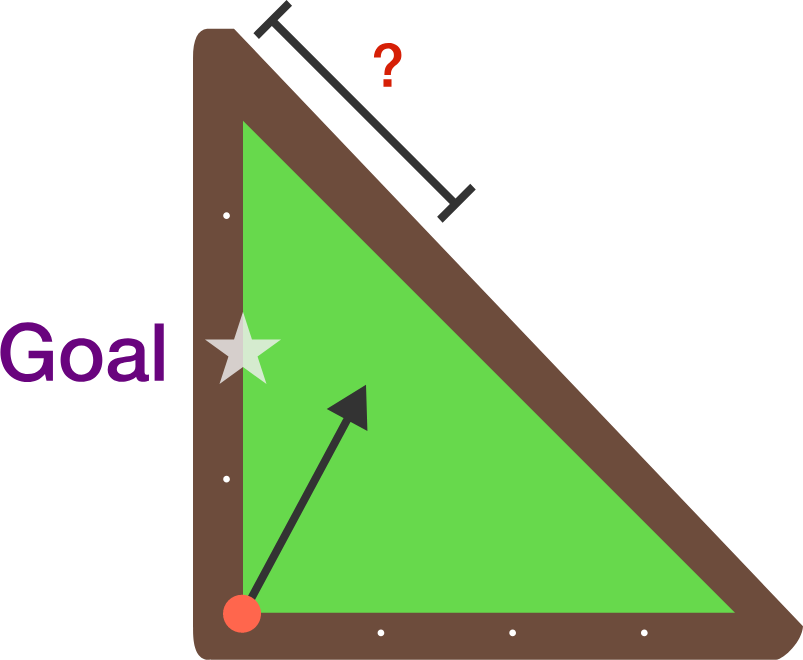# Pool Shark #2If you have a pool table as shown shaped like an isosceles right triangle, with a ball that starts at the right angle, where should you aim at on the hypotenuse so after one bounce the ball hits the midpoint of the left-hand side of the table?

This problem is part of the Brilliant.org Open Problems Group. The end goal for each open problem is to find a solution, and maybe publish it if it's a nice enough result! You can read about the unsolved billiards problem that this one is related to here.

Assume geometric purity: the "ball" has no mass, the "ball" and "hole" are considered single points, and the ball travels straight.

×